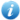English  |  正體中文  |  简体中文  |  Items with full text/Total items : 70585/70585 (100%) Visitors : 23070766      Online Users : 287
 Scope All of NCUIR 理學院    數學研究所       --博碩士論文Tips: please add "double quotation mark" for query phrases to get precise resultsplease goto advance search for comprehansive author search Adv. Search
 Home ‧ Login ‧ Upload ‧ Help ‧ About ‧ AdministerNCU Institutional Repository > 理學院 > 數學研究所 > 博碩士論文 >  Item 987654321/7785

 Please use this identifier to cite or link to this item: `http://ir.lib.ncu.edu.tw/handle/987654321/7785`

 Title: 圖形的線性蔭度及星形蔭度;Linear Arboricity and Star Arboricity of Graphs Authors: 黃文婷;Wen-Ting Huang Contributors: 數學研究所 Keywords: 完全三部圖;迴路的次方;完全多部圖;不限路徑數;星形蔭度;線性蔭度;complete tripartite graph;complete multipartite graph;power of cycle;linear arboricity;star arboricity;unrestricted path number Date: 2003-07-02 Issue Date: 2009-09-22 11:05:21 (UTC+8) Publisher: 國立中央大學圖書館 Abstract: 一個線性森林(linear forest)是一個森林(forest)，其中每一個部分(component)是一條路徑(path)。令G是一個圖(graph)。G的蔭度(arbori- city)，用a(G)來表示，是分解G的邊所需要的最少的森林數。G的線性蔭度(linear arboricity)，用la(G)來表示，是分解G的邊所需要的最少的線性森林數。G的不限路徑數(unrestricted path number)，是覆蓋G的邊所需要的最少的路徑數。在第二章中，我們得到規則完全r部圖(regular complete r-partite graphs)的線性蔭度及不限路徑數。在第三章中，我們得到完全三部圖(complete tripartite graphs)的線性蔭度。 對一個圖G一個正整數k，令G^k代表V(G)=V(G^k)且E(G^k)={uv:u,v in V(G), u e u, d_G(u,v)le k}的圖，其中d_G(u,v)代表在G中u和v的距離。我們稱G^k為G的k次方。 一個每個部分(component)都是星形(star)的森林稱為星林(star for- est )。G的星形蔭度(star arboricity)，用sa(G)來表示，是分解G的邊所需要的最少的星林數。在第四章中，我們得到迴路(cycle)的次方的星形蔭度。在第五章中我們進一步提出了一些相關的問題。 Let G be a graph. The unrestricted path number of G, denoted by ho(G), is the minimum number of paths needed to cover the edges of G. The arboricity of G, denoted by a(G), is the minimum number of forests needed to decompose the edges of G. A linear forest is a forest in which each component is a path. The linear arboricity of G, denoted by la(G), is the minimum number of linear forests needed to decompose the edges of G. In Chapter 2, we study the linear arboricity and unrestricted path number of regular complete r-partite graphs. Let K_{r imes n} denote the complete r-partite graph such that each part has n\$vertices, For rge 2, we obtain a(K_{r imes n})= la(K_{r imes n})= ho (K_{r imes n})=lceil frac{(r-1)n+1}{2} ceil. In Chapter 3, we study the linear arboricity of complete tripartite graphs. Suppose n_1ge n_2ge n_3, we obtain la(K_{n_1, n_2, n_3})=n_1+1 if n_1=n_2=n_3, and la(K_{n_1, n_2, n_3})=lceilfrac{n_1+n_2}{2} ceil otherwise. For a graph G and a positive integer k, let G^k denote the graph with V(G^k)=V(G) and E(G^k)={uv : u, vin V(G), u e v, d_G(u, v)le k} where d_G(u, v) denotes the distance between u and v in G. We call G^k the k-th power of G. A star forest is a forest in which each component is a star. The star arboricity of a graph G, denoted by sa(G), is the minimum number of star forests needed to decompose the edges of G. In Chapter 4, we study the star arboricity of power of cycles. Suppose that k, n are integers such that 2le kle lfloorfrac{n}{2} floor-1, we obtain sa(C_n^k)=k+1 if n=0(mod k+1), and sa(C_n^k)=k+2 otherwise In Chapter 5, some problems are proposed for further investigations. Appears in Collections: [數學研究所] 博碩士論文

Files in This Item:

File SizeFormat